Study P3 Mathematics Length, Mass and Volume 1 - Geniebook# Length, Mass And Volume

## Measuring Length

To measure the length of an object, we will usually use a ruler. In most occasions, we will place the object at the starting point and see where it ends, and that would be the length of the object.

It is also possible to start measuring from any point. In these cases, we will have to do subtraction to find the length of the object.

Question 1:

Look at the diagram below.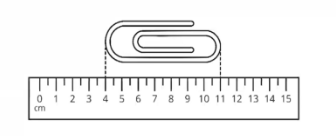The length of the paper clip is __________ $$\text{ cm }$$.

Solution:

Length of the paper clip

$$=$$ Length at end point - Length at start point

$$= 11 \text{ cm } - 4 \text{ cm }$$

$$= 7 \text{ cm }$$

$$7 \text{ cm }$$

Question 2:

Look at the diagram below.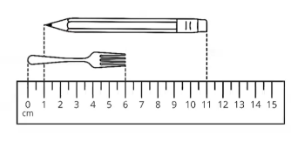The pencil is __________ $$\text{ cm }$$ longer than the fork.

Solution:

Length of the pencil

$$=$$ Length at end point $$-$$ Length at start point

$$= 11 \text{ cm } - 1 \text{ cm }$$

$$= 10 \text{ cm }$$

Length of the fork

$$=$$ Length at end point $$-$$ Length at start point

$$= 6 \text{ cm } - 0 \text{ cm }$$

$$= 6 \text{ cm }$$

Difference in length between the pencil and the fork

$$= 10 \text{ cm } - 6 \text{ cm }$$

$$= 4 \text{ cm }$$

$$4 \text{ cm }$$

Question 3:

Look at the diagram below.The total length of Sticks A, B and C is __________ $$\text{ cm }$$.

1. 9
2. 12
3. 15
4. 20

Solution:

Length of Stick A

$$=$$ Length at end point $$-$$ Length at start point

$$= 9 \text{ cm } - 1 \text{ cm }$$

$$= 8 \text{ cm }$$

Length of Stick B

= Length at end point $$-$$ Length at start point

$$= 7 \text{ cm } - 0 \text{ cm }$$

$$= 7 \text{ cm }$$

Length of Stick C

$$=$$ Length at end point $$-$$ Length at start point

$$= 8 \text{ cm } - 3 \text{ cm }$$

$$= 5 \text{ cm }$$

Total length of Sticks A, B and C

$$= 8 \text{ cm } + 7 \text{ cm } + 5 \text{ cm }$$

$$= 20 \text{ cm }$$

(4) $$20 \text{ cm }$$

Question 4:

Look at the diagram below.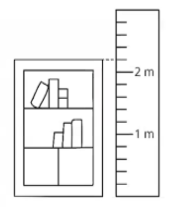What is the height of the bookshelf?

1. 200$$\text{ cm }$$
2. 210$$\text{ cm }$$
3. 220$$\text{ cm }$$
4. 240$$\text{ cm }$$

Solution:

$$1 \text{ m }= 100 \text{ cm }$$

Length represented by 5 intervals $$= 100 \text{ cm }$$

Length represented by 1 interval

$$= 100 \div 5\text{ cm }$$

$$= 20 \text{ cm }$$

The height of the cupboard is 1 interval above $$2 \text{ m}$$

Height of the bookshelf

$$= 2 \text{ m } 20 \text{ cm }$$

$$= 220 \text{ cm }$$

(3) $$220\text{ cm }$$

## Measuring Mass

To measure the mass of an object, we usually use a weighing scale. To find the mass of the object, we have to first find the value of each interval. From there, we will be able to determine the mass of the object by looking at which interval the arrow is pointing to.

Question 1:

Look at the diagram below.The mass of the bag of rice is __________.

Solution:

Mass represented by 10 intervals $$= 1000 \text{ g }$$

Mass represented by 1 interval

$$= 1000 \text{ g } \div 10$$

$$= 100 \text{ g }$$

The mass of the bag of rice is 4 intervals after $$4 \text{ kg }$$

Mass of the bag of rice $$= 4 \text{ kg } 400 \text{ g }$$

$$4 \text{ kg } 400 \text{ g }$$ or $$4400 \text{ g }$$.

Question 2:

Look at the diagram below.What is the mass of the grapes?

1. 356$$\text{ g }$$
2. 360$$\text{ g }$$
3. 600$$\text{ g }$$
4. 3600$$\text{ kg }$$

Solution:

Mass represented by 10 intervals $$= 100 \text{ g }$$

Mass represented by 1 interval

$$= 100 \text{ g } \div 10$$

$$= 10 \text{ g }$$

The mass of the grapes is 6 intervals after $$300 \text{ g }$$

Mass of grapes $$= 360 \text{ g }$$

(2) $$360\text{ g }$$

Question 3:

Look at the diagram below.The total mass of the grapes and bananas is __________.

Solution:

Mass represented by 10 intervals $$= 100 \text{ g }$$

Mass represented by 1 interval

$$= 100 \text{ g} \div 10$$

$$= 10 \text{ g }$$

The mass of grapes is 5 intervals after $$300 \text{ g }$$

Mass of the grapes $$= 350 \text{ g }$$

The mass of bananas is 3 intervals after $$200 \text{ g }$$

Mass of the bananas $$= 230\text{ g}$$

Total mass of the grapes and bananas

$$= 350 \text{ g } + 230 \text{ g }$$

$$= 580 \text{ g }$$

$$580 \text{ g }$$

Question 4:

Look at the diagram below.

The mass of the big ball is $$39 \text{ g }$$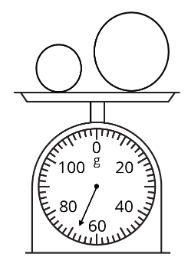What is the mass of the small ball?

1. 29$$\text{ g }$$
2. 30$$\text{ g }$$
3. 31$$\text{ g }$$
4. 32$$\text{ g }$$

Solution:

Mass represented by 10 intervals $$= 20 \text{ g }$$

Mass represented by 1 interval

$$= 20 \text{ g} \div 10$$

$$= 2\text{ g }$$

The total mass of the big ball and the small ball is 4 intervals after $$60 \text{ g }$$

Total mass of the big ball and small ball $$= 68 \text{ g }$$

Mass of small ball

$$= 68 \text{ g} - 39 \text{ g }$$

$$= 29\text{ g }$$

(1) $$29 \text{ g }$$

Question 5:

Look at the diagram below.

4 identical apples are placed on a weighing scale.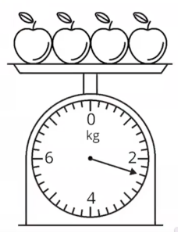The mass of 1 apple is __________.

Solution:

Mass represented by 10 intervals $$= 2000 \text{ g }$$

Mass represented by 1 interval

$$= 2000\text{ g} \div 10$$

$$= 200\text{ g }$$

The mass of 4 apples is 2 intervals after $$2 \text{ kg}$$

Mass of 4 apples $$= 2400\text{ g }$$

Mass of 1 apple

$$= 2400 \text{ g} \div 4$$

$$= 600 \text{ g }$$

The mass of 1 apple is $$600\text{ g }$$.

$$600\text{ g }$$

Question 6:

Look at the diagram below.

The weighing scale shows the mass of a basket and 5 identical apples.

The mass of the basket is $$150\text{ g }$$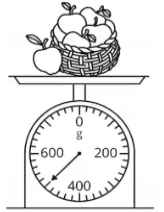What is the mass of 1 apple?

1. 50$$\text{ g }$$
2. 70$$\text{ g }$$
3. 350$$\text{ g }$$
4. 500$$\text{ g }$$

Solution:

Mass of the basket and 5 apples $$= 500 \text{ g }$$

Mass of 5 apples

$$= 500 \text{ g} - 150 \text{ g }$$

$$= 350\text{ g }$$

Mass of 1 apple

$$= 350 \text{ g} \div 5$$

$$= 70 \text{ g }$$

$$70\text{ g }$$

Question 7:

Look at the diagram below.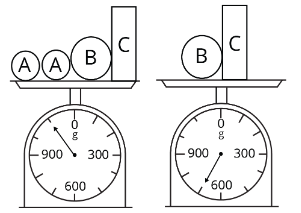What is the mass of object A?

1. 200$$\text{ g }$$
2. 400$$\text{ g }$$
3. 700$$\text{ g }$$
4. 1100$$\text{ g }$$

Solution:

Mass of 2 Object A, Object B and Object C $$= 1100 \text{ g }$$

Mass of Object B and Object C $$= 700 \text{ g }$$

Mass of 2 Objects A

$$= 1100 \text{ g} - 700 \text{ g }$$

$$= 400 \text{ g }$$

Mass of Object A

$$= 400 \text{ g} \div 2$$

$$= 200 \text{ g }$$

(1) $$200\text{ g }$$

## Measuring Volume

To measure the volume of water, we usually use a beaker or a measuring cylinder.

To find the volume of water, we have to first find the value of each interval. From there, we will be able to determine the volume of water by looking at the water level.

Question 1:

Look at the diagram below.The volume of the water in the beaker is __________.

Solution:

Volume represented by 5 intervals $$= 1000 \text{ mℓ }$$
Volume represented by 1 interval \begin{align}\\[2ex] &= 1000 \text{ mℓ} \div 5 \\[2ex] &= 200 \text{ mℓ} \end{align}a
3rd interval\begin{align}\\[2ex] &= 3 \div 200 \text{ mℓ } \\[2ex] &= 600 \text{ mℓ } \end{align}

$$600 \text{ mℓ }$$

Question 2:

Look at the diagram below.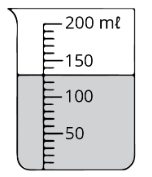What is the volume of the water in the beaker?

1. 103$$\text{ mℓ }$$
2. 130$$\text{ mℓ }$$
3. 133$$\text{ mℓ }$$
4. 1300$$\text{ mℓ }$$

Solution:

Volume represented by 5 intervals $$= 50 \text{ mℓ }$$

Volume represented by 1 interval $$= 50 \text{ mℓ} \div 5$$

Volume represented by 1 interval $$= 10 \text{ mℓ }$$

The volume of water in the beaker is 3 intervals after $$100 \text{ mℓ }$$.

Volume of water in the beaker $$= 130 \text{ mℓ }$$

(2) $$130\text{ mℓ }$$

Question 3:

Look at the diagram below.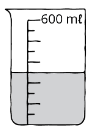What is the volume of the water in the beaker?

1. 250$$\text{ mℓ }$$
2. 300$$\text{ mℓ }$$
3. 450$$\text{ mℓ }$$
4. 500$$\text{ mℓ }$$

Solution:

Volume represented by 10 intervals $$= 600 \text{ mℓ }$$

Volume represented by 1 interval $$= 600 \text{ mℓ } \div 10$$

Volume represented by 1 interval $$= 60 \text{ mℓ }$$
Volume of water in the beaker\begin{align}\\[2ex] &= 60 \text{ mℓ} \times 5\\[2ex] &= 300 \text{ mℓ } \end{align}

(2) $$300\text{ mℓ }$$

Continue Learning
Volume Money
Mass Fractions
Addition And Subtraction Whole Numbers 1
Whole Numbers 3 Whole Numbers 6
Bar Graphs Angles
Multiplication And Division Length, Mass And Volume
Length Area And Perimeter
Parallel And Perpendicular LinesPrimaryPrimary 1Primary 2Primary 3EnglishMaths
Volume
Money
Mass
Fractions
Whole Numbers 1
Whole Numbers 3
Whole Numbers 6
Bar Graphs
Angles
Multiplication and Division
Length, Mass And Volume
Length
Area and Perimeter
Parallel And Perpendicular LinesSciencePrimary 4Primary 5Primary 6SecondarySecondary 1Secondary 2Secondary 3Secondary 4